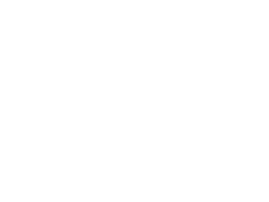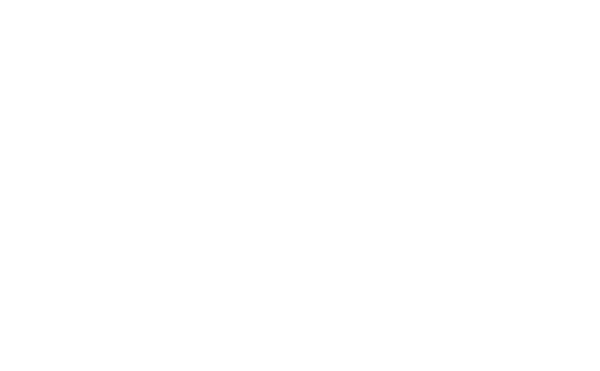# Linear Inequalities Questions and Answers - Form 1 Topical Mathematics

Questions

1. Determine the inequalities that represent and satisfies the unshaded region (3 mks)2. The diagram below shows the graphs of y = 3/10 x – 3/2, 5x + 6y = 3 and x = 2By shading the unwanted region, determine and label the region
R that satisfies the three inequalities; y 3/10 x – 3/2, 5x + 6y 30 and x 2
3. The cost of 7 shirts and 3 pairs of trousers is shs. 2950 while that of 5 pairs of trousers and 3 shirts is less by 200. How much will Dan pay for 2 shirts and 2 pairs of trousers?
4. Mr. Wafula went to the supermarket and bought two biros and five pencils at sh.120. Whereas three biros and two pencils cost him sh.114. Find the cost of each biros and pencils
5. A father is twice as old as his son now. Ten years ago, the ratio of their ages was 5:2. Find their present ages
6. List the integral values of x which satisfy the inequalities below:-
2x + 21
15 – 2x ≥ x + 6
7. Find the equation of a line which passes through (-1, -4) and is perpendicular to the line: y + 2x – 4 = 0
8. John bought two shirts and three pairs of trousers at Kshs. 1750. If he had bought three shirts and two pairs of trousers, he would have saved Kshs. 250. Find the cost of a shirt and a trouser.
9. Express the recurring decimal 3.81 as an improper fraction and hence as a mixed number
10. Karani bought 4 pencils and 6 biro pens for shs.66 and Mary bought 2 pencils and 5 biro pens for shs.51
1. Find the price of each item
2. Ondieki spent shs.228 to buy the same type of pencils and biro pens. If the number of biro pens he bought were 4 more than the number of pencils, find the number of pencils he bought
11. Two consecutive odd numbers are such that the difference of twice the larger number and twice the smaller number is 21.Find the product of the numbers
12. The size of an interior angle of a regular polygon is 3xo while its exterior angle is (x-20)oFind the number of sides of the polygon
13. Five shirts and four pairs of trousers cost a total of shs.6160. Three similar shirts and a pair of trouser cost shs.2800. Find the cost of four shirts and two pairs of trousers
14. Two pairs of trousers and three shirts costs a total of shs.390. Five such pairs of trousers and two shirts cost a total of shs.810. Find the price of a pair of trouser and a shirt

1. Equations of the lines enclosing the shaded area
x=1
y=0
gradient of the line = We pick any two arbitrary points on the third line (0,3); (3,0)
= 0 − 3/3 − 0 = −3/3 = −1
To find the equation of the line in the format y=mx+c we pick another point (x,y) and pair it with any of the two points earlier chosen
(x − 3)/(y − 0) = − 1
x − 3 = − y
in the form y=mx + c
y= −x + 3
Therefore the inequalities are
x ≥ 1
y ≥ 0
y < −x+3
2. The diagram below shows the graphs of
Y = 3/10 x −3/2 , 5x + 6y = 30 and x = 2
By shading the unwanted region, determine and label the region R that satisfies the three inequalities;
Y
3/10 x -3/2, 5x + 6y 30 and x 2 (2 mks)
L
1 y = 3/10 x -3/2 at (0, 0)
0
2 *

Picking P(0,0)
0
≥ -3/2
L
2  5x + 6y = 30
At (0, 0) 5x + 6y
30
0
30 *
3. 7s + 3t = 2950 ………………….(i) x 5
3s + 5t = 2750 …………………..(ii) x 3
35s + 15t = 14750
(9s + 15t = 8250)/(26s = 6500)
s = 250
t = 2750 − 3(250)/5 = 400
2t + 2s = 2(400) + 2(250)
= shs. 1,300
4. Let the cost of a biro be b
Pencil be p
2b + 5p = 120 x 3
3b + 2p = 114 x 2
6b + 15p = 360
(6b + 4p = 228)/(11p = 132)
P = 121
2b + 60 = 120
2b = 60
b = 30
The cost of 1 biro is 30/=
The cost of 1 pencil is 12/=
5. Let son’s present age be n yrs
Father’s age is 2n yrs
Ten years ago: son’s age →
n −10
Father’s age →
2n − 10
Son’s present age = 30yrs
Father’s present age = 2x 30 = 60yrs
6. 2x + 21 > 15 − 2x
4x 0.6

15 − 2x ≥
x + 6
-3 x ≥ -9

x > -1 ½
⇒ -1 ½ x 3
Values are −1, 0, 1, 2, 3.
7. y = -2x + 4
gradient of h line is ½
Equation (y + 4)/(x + 1) = ½

2y + 8 = x + 1
2y − x + 7 = 0
8. 2s + 3t = 1750
3s + 2t = 1500
4s + 6t = 3500
9s + 6t = 4500
2t = 1500 − 600
t = 450
5s = 1000
s = 200
Shirt = sh 200
Trouser = sh 450
1. Let cost of pencils be x and biro pens to be y
4x + 6y = 66
2x + 5y = 51

4x + 6y = 66
4x + 10y = 102
4y = 96
y = 24
Correct substitution
x = 3
Pencils = shs.9
Biro pens = 3
2. (b) 9p + 3b = 228...(i)
b − y = 4
b = 4 + r ...........(ii)
substituting for b in ..........(i)
p
+ 5p − 288 = 0
p = (-5 ±
√25 − 4 x 1 x -228)/(2 x 1)
P = 13 (to the nearest whole no.)
b= 4+ 13 = 17
9. 3x − 2 (x + 2) = 21
X = 25
Large No = 25 + 2 = 27

product = 25 x 27 = 695
10. x − 20 + 3x = 180oC
4x = 200
x = 50

Attempt to get x by using l+e = 180o
e = (2n-4)90/n
number of sides

11. 5x + 4y = 6160
4(3x + y = 2800
-7x = −5040
x = 720
y = 640
4(720) + 2(640) = 4160
12. 2x + 3y = 390
5x + 2y = 810
15x + 6y = 2430
(4x + 6y = 780)/(11x = 1650)
x = 150
A pair of trouser =sh150
A shirt = sh30

• ✔ To read offline at any time.
• ✔ To Print at your convenience
• ✔ Share Easily with Friends / Students